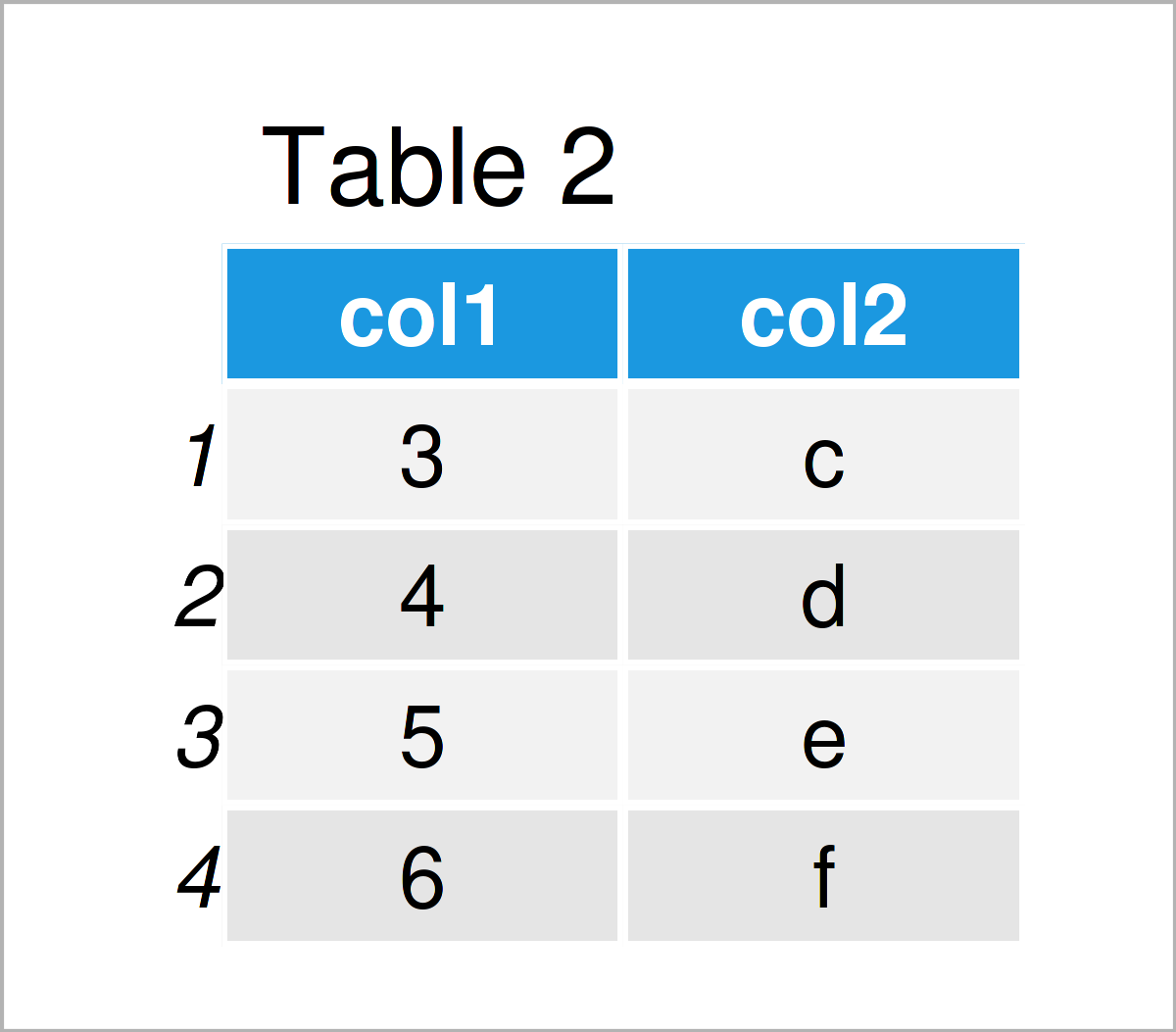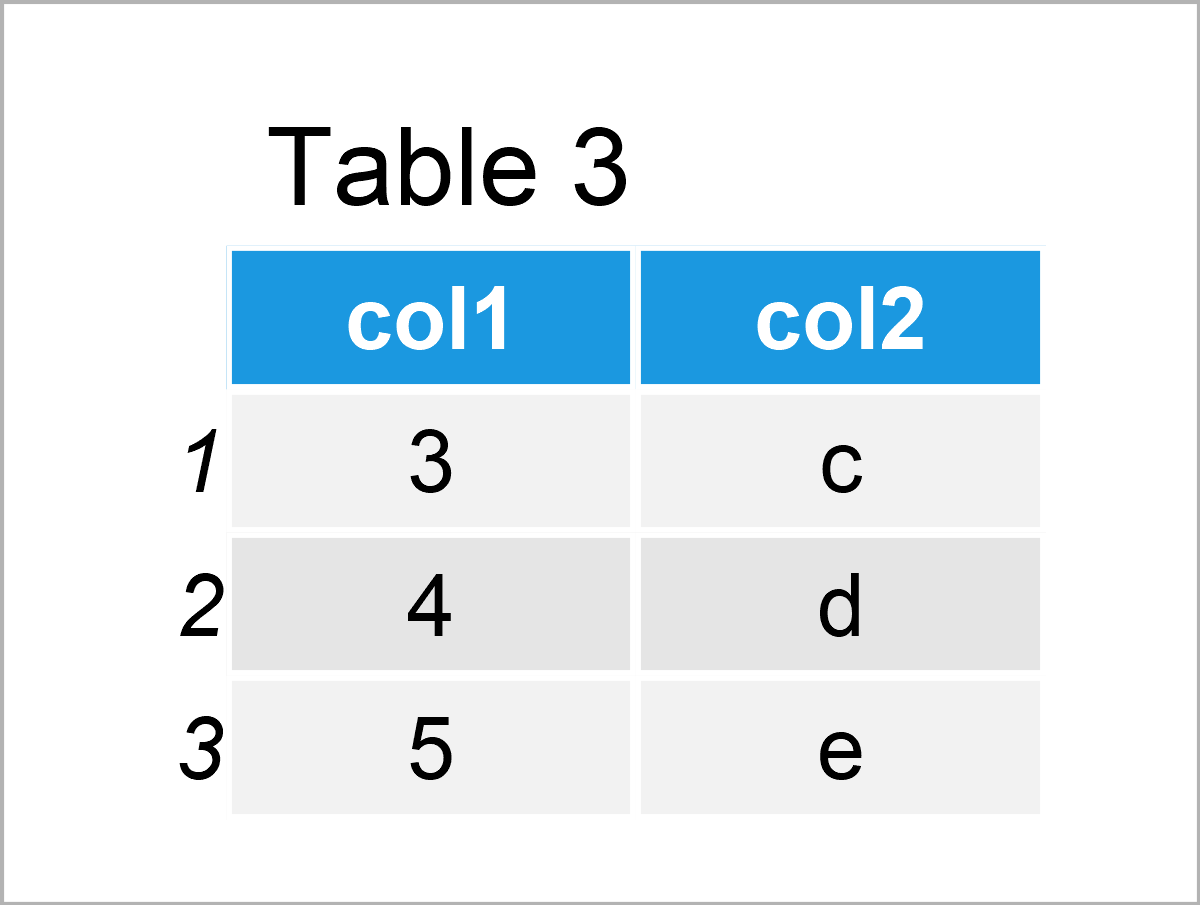# intersect Function in R (2 Examples)

In this tutorial you’ll learn how to return the intersection of two data objects using an intersect() function in R programming.

Note: There are several different packages available that provide a function called intersect (e.g. Base R, dplyr, lubridate, and generics). Depending on what you want to do, those functions may have to be applied differently. For that reason, it is important to specify the package you want to use when calculating intersections.

Anyway, let’s move on with the tutorial. This tutorial consists of the following topics:

So now the part you have been waiting for – the R code…

## Example 1: Apply intersect() Function to Vector Objects

Example 1 demonstrates how to return the intersection of two vector objects. First, we have to create some example vectors:

```x1 <- letters[1:5]                                # Create first vector object
x1                                                # Print first vector object
#  "a" "b" "c" "d" "e"```
```x2 <- letters[3:6]                                # Create second vector object
x2                                                # Print second vector object
#  "c" "d" "e" "f"```

As you can see based on the previous outputs of the RStudio console, we have created two vectors containing character letters.

If we want to find the elements that are contained in both vectors, we can apply the intersect function provided by the basic installation of the R programming language as shown below:

```x1_x2_inter <- base::intersect(x1, x2)            # Apply intersect function to vectors
x1_x2_inter                                       # Print intersection of vectors
#  "c" "d" "e"```

The intersection of our two vectors has been returned to the RStudio console, i.e. the characters “c”, “d”, and “e”.

## Example 2: Apply intersect() Function to Data Frames

The following syntax demonstrates how to identify the row-wise intersection between two data frames.

Let’s create some example data:

```data1 <- data.frame(col1 = 1:5,                   # Create first data frame
col2 = letters[1:5])
data1                                             # Print first data frame```The output of the previous R programming code is shown in Table 1: We have created a data frame with two columns.

Let’s create another data frame:

```data2 <- data.frame(col1 = 3:6,                   # Create second data frame
col2 = letters[3:6])
data2                                             # Print second data frame```In Table 2 it is shown that we have created a second data frame with the same column names by executing the previous syntax.

Next, we can use the intersect function of the dplyr package (note that dplyr has exported this function from the generics package) to return row-wise intersections:

```data_1_2_inter <- dplyr::intersect(data1, data2)  # Apply intersect function to data frames
data_1_2_inter                                    # Print intersection of data frames```In Table 3 it is shown that we have created a data frame containing all rows that exist in both of our input data frames with the previous R programming syntax.

## Video, Further Resources & Summary

I have recently released a video on the Statistics Globe YouTube channel, which explains the R programming syntax of the present article. You can find the video below.

Please accept YouTube cookies to play this video. By accepting you will be accessing content from YouTube, a service provided by an external third party.If you accept this notice, your choice will be saved and the page will refresh.

In addition, you may have a look at the related RStudio articles on this homepage. You can find some interesting tutorials below:

You have learned in this tutorial how to apply the intersect function in R. In case you have further questions, let me know in the comments section. Furthermore, please subscribe to my email newsletter in order to receive regular updates on new tutorials.

Subscribe to the Statistics Globe Newsletter